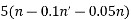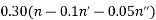Status: Active

# DETERMINATION OF VOLATILE ACIDITY IN GRAPE JUICE, RECONSTITUTED GRAPE JUICE, CONCENTRATED GRAPE JUICE, AND GRAPE NECTAR

Type IV method

THE GENERAL ASSEMBLY,

IN VIEW of Article 2, paragraph iv of the Agreement of 3 April 2001 establishing the International Organisation of Vine and Wine,

AT THE PROPOSAL of the "Methods of Analysis" Sub-Commission,

CONSIDERING that for grape juice, reconstituted grape juice, concentrated grape juice, and grape nectar is proposed the application of the Method OIV-MA-AS313-02 (Volatile Acidity) of the Compendium of International Methods of Analysis of Wines and Musts,

CONSIDERING that for concentrated grape juice a simple dilution need to be done before the analysis,

DECIDES to add the following method:

DETERMINATION OF VOLATILE ACIDITY IN GRAPE JUICE, RECONSTITUTED GRAPE JUICE, CONCENTRATED GRAPE JUICE, AND GRAPE NECTAR

Type IV method

1.          Scope

This method applies to volatile acid determination in grape juice, reconstituted grape juice, concentrated grape juice, and grape nectar by titration, at the indicated concentrations ranging from 0.11 up to 1.09 g/L in acetic acid.

1.          Definition

The volatile acidity is derived from the acids of the acetic series present in grape juice, reconstituted grape juice, concentrated grape juice, and grape nectar in its free state and combined as salts.

1.          Principle

Volatile acids are separated from the grape juice, reconstituted grape juice, concentrated grape juice*, and grape nectar by steam distillation and titrated using sodium hydroxide solution of known concentration. The acidity of free and combined sulfur dioxide distilled under these conditions should be subtracted from the acidity of the distillate. The acidity of any sorbic acid, which may have been added to the grape juice, reconstituted grape juice, concentrated grape juice, and grape nectar must also be subtracted.

Note: Part of the salicylic acid used to stabilize the grape juices before analysis is present in the distillate. This must be determined and subtracted from the acidity as show on section 7.3.

1.           Reagents and materials

4.1.        Reagents

4.1.1.                        Tartaric acid 99.5 % (C4H6O6) CAS [87-69-4].

4.1.2.                        Sodium hydroxide (NaOH) CAS [1310-73-2] solution 0.1 M.

4.1.3.                        Phenolphthalein 98 % (‎C20H14O4) CAS [77-09-8].

4.1.4.                        Hydrochloric acid 37 % (HCl) CAS [7647-01-0].

4.1.5.                        Iodine (I2) CAS [7553-56-2] solution 0.005 M.

4.1.6.                        Potassium iodide 99.0 % (KI) CAS [7681-11-0].

4.1.7.                        Starch (C6H10O5)n CAS [9005-84-9].

4.1.8.                        Sodium tetraborate 99.5 % (Na2B4O7.10H2O) CAS [1303-96-4].

4.1.9.                        Acetic acid 99.8 % (CH₃COOH) CAS [64-19-7] solution 0.1 M.

4.1.10.                    Lactic acid 85 % (C3H6O3) CAS [50-21-5].

4.1.11.                    Sodium chloride 99.0 % (NaCl) [7647-14-5].

4.1.12.                    Sodium thiosulfate 99.5 % (Na2S2O3.5H2O) CAS [10102-17-7] solution 0.1 M or a commercial solution.

4.1.13.                    Iron (III) ammonium sulfate 99.0 % (Fe(NH4)2(SO4)2.12H2O) CAS [7783-83-7] 10 % (m/v).

4.1.14.                    Sodium salicylate 99.5 % (NaC7H5O3) CAS [54-21-7] solution 0.01 M.

4.1.15.                    Ethanol 96 % (C2H6O) CAS [64-17-5].

4.1.16.                    Water Type 1 (EN ISO 3696) or equivalente ultrapure.

4.2.        Preparation of solutions

4.2.1.                        Phenolphthalein solution 1 % m/v

Mix 1 g of phenolphthalein (4.1.3) in 100 mL of ethanol (4.1.15).

Commercial solution can be used.

4.2.2.                        Starch indicator solution 5 g/L

Mix 5 g of starch (4.1.7) with about 500 mL of water (4.1.16). Bring to the boiling point, stirring continuously and boil for 10 min. Add 200 g of sodium chloride (4.1.11). When cool, complete to one liter of water (4.1.16).

4.2.3.                        Saturated solution of sodium tetraborate about 55 g/L

Mix 55 g of sodium tetraborate (4.1.8) in one liter of water (4.1.16).

4.2.4.                        Lactic acid solution 0.1 M - 90 g/L

Dilute 100 mL of lactic acid (4.1.10) in 400 mL of water (4.1.16). As an example, this solution is heated in an evaporating dish over a boiling water bath for four hours, topping up the volume occasionally with water (4.1.16). After cooling, complete to a liter of water (4.1.16). Titrate the lactic acid in 10 mL of sodium hydroxide solution (4.1.2). Adjust the concentration of the lactic acid solution for 90 g/L.

1.          Apparatus

5.1.        Steam distillation apparatus consisting of:

• a steam generator; the steam must be free of carbon dioxide;
• a flask with a steam pipe;
• a distillation column;
• a condenser.

This equipment must pass the three following tests:

(a) place 20 mL of distilled water free of CO2 in the flask. Collect 250 mL of the distillate and add to it 0.1 mL of sodium hydroxide solution (4.1.2) and two drops of phenolphthalein solution (4.2.1). The pink coloration must be stable for at least 10 s (i.e. steam to be free of carbon dioxide);

(b) place 20 mL acetic acid solution (4.1.9), in the flask. Collect 250 mL of the distillate. Titrate with the sodium hydroxide solution (4.1.2) and two drops of phenolphthalein solution (4.2.1). The volume of the titer must be at least 19.9 mL (i.e. at least 99.5 % of the acetic acid entrained with the steam;

(c) place 20 mL lactic acid solution (4.2.4), in the flask. Collect 250 mL of the distillate and titrate the acid with the sodium hydroxide solution (4.1.2) and two drops of phenolphthalein solution (4.2.1). The volume of sodium hydroxide solution (4.1.2) added must be less than or equal to 1.0 mL (i.e. not more than 0.5 % of lactic acid is distilled).

Any apparatus (automated or not) or procedure, which passes these tests satisfactorily, fulfils the requirements of official international apparatus or procedures.

5.2.        Volumetric material

5.3.        Analytical balance verified and calibrated

5.4.        Volumetric pipettes of 10 mL and 20 mL

5.5.        Burette

5.6.        Water bath

1.         Procedure

6.1.        Preparation of sample

For gasified grape juices to eliminate CO2:

place about 50 mL of grape juice, reconstituted grape juice, and grape nectar in a vacuum flask; apply vacuum to the flask with the water pump for one to two minutes while shaking continuously. Other CO2 elimination systems may be used if the CO2 elimination is guaranteed.

6.2.        Steam distillation

Place 20 mL of grape juice, reconstituted grape juice, concentrated grape juice* and grape nectar into the flask. Add about 0.5 g of tartaric acid (4.1.1). Collect at least 250 mL of the distillate.

* The concentrated grape juice must be diluted (200 g to 500 mL, for example) and multiply the result by the dilution factor (F = 2.5, in this case).

6.3.        Titration

Titrate with the sodium hydroxide solution (4.1.2), using two drops of phenolphthalein solution (4.2.1) as indicator. Let n mL be the volume of sodium hydroxide solution (4.1.2) used. Add four drops the hydrochloric acid (4.1.4) dilute 1/4 (v/v) with water (4.1.16), 2 mL of starch solution (4.2.2) and a few crystals of potassium iodide (4.1.6). Titrate the free sulfur dioxide with the iodine solution (4.1.5). Let n' mL be the volume used. Add the saturated solution of sodium tetraborate (4.2.3) until the pink coloration reappears. Titrate the combined sulfur dioxide with the iodine solution (4.1.5). Let n" mL be the volume used.

1.         Calculation (Results)

7.1.        Method of calculation

The volatile acidity, expressed in milliequivalents per liter to one decimal place, is given by:The volatile acidity, expressed in grams of acetic acid per liter to two decimal places, is given by:n = volume (mL) of sodium hydroxide solution (4.1.2) used.

n' = volume (mL) of iodine solution (4.1.5) used on the titration of the free sulfur dioxide.

n" = volume (mL) of iodine solution (4.1.5) used on the titration of the combined sulfur dioxide.

Note: In the case of the concentrated grape juice multiply the result by the dilution factor (F).

7.2.        Samples with sorbic acid present

Since 96% of sorbic acid is steam distilled with a distillate volume of 250 mL, its acidity must be subtracted from the volatile acidity, knowing that 100 mg of sorbic acid corresponds to an acidity of 0.89 milliequivalents or 0.053 g of acetic acid and knowing the concentration of sorbic acid in mg/L as determined by other methods.

7.3.        Samples with salicylic acid present

7.3.1.                        Identification of salicylic acid in the volatile acidity distillate

Immediately after the determination of the volatile acidity and the correction for free and combined sulfur dioxide, introduce into a conical flask 0.5 mL hydrochloric acid (4.1.4), 3 mL of the sodium thiosulfate solution (4.1.12) and 1 mL of the iron (III) ammonium sulfate solution (4.1.13). If salicylic acid is present, a violet coloration appears.

7.3.2.                        Determination the salicylic acid

On the above conical flask, indicate the volume of the distillate by a reference mark. Empty and rinse the flask. Subject a new test sample of 20 mL grape juice or reconstituted grape juice or concentrated grape juice (see section 6.2) or nectar to steam distillation and collect the distillate in the conical flask up to the reference mark. Ensure that the same volume of distillate is collected as in section 7.3.3.  Add 0.3 mL hydrochloric acid (4.1.4) and 1 mL of the iron (III) ammonium sulfate solution (4.1.13). The contents of the conical flask turn violet. Into a conical flask identical to that carrying the reference mark, introduce distilled water (4.1.16) up to the same level as that of the distillate. Add 0.3 mL hydrochloric acid (4.1.4) and 1 mL of the iron (III) ammonium sulfate solution (4.1.13). Titrate the distillate water in the conical frask with sodium salicylate solution (4.1.14) until the violet coloration obtained has the same intensity as that of the conical flask containing the wine distillate. Let n''' mL be the volume of solution added from the burette.

7.3.3.                        Correction to the volatile acidity

Subtract the volume 0.1 x n''' mL from the volume n mL of sodium hydroxide solution (4.1.2) used to titrate the acidity of the distillate during the determination of volatile acidity.

1.         Indicative characteristics of the method

A validation study with grape juice was carried out for the purpose of assessing the suitability of the method for the matrices in question, taking into account linearity, limits of detection and quantification and the accuracy of the method. The latter parameter was determined by defining the levels of precision and trueness of the method.

8.1.        Linearity of the method

The method proved to be linear within the ranges 0.11 g/L – 1.09 g/L shown in the Table 1.

8.2.        Limit of detection and limit of quantification

The detection limit (LD) and limit of quantification (LoQ) were calculated from 7 analytical  repetitions of an 0.11 g/L aqueous acetic acid solution and is equal to 3 standard deviations for the LD and 10 standard deviations for the LoQ (Table 1).

8.3.        Precision of the method

The parameters taken into account were repeatability and reproducibility. Table 1 shows the values of these parameters. The repeatability was expressed as Relative Standard Deviation (%RSD) of measurements repeated in different concentrations found for grape juice. And the reproducibility was expressed as the average of the Relative Standard Deviation (%RSD) of measurements made of the same grape juice sample, by different operators.

8.4.        Trueness of the method

The percentage recovery was determined using a grape juice sample spiked in 6 different levels of acetic acid, ranging from 0.11 g/L to 1.09 g/L.

Table 1. Characteristics of the method

 Linearity range (g/L of acetic acid) Correlation coefficient (r2) LD  (g/L of acetic acid) LoQ (g/L of acetic acid) Repeatability (n = 7) %RSD Reproducibility (n = 7) %RSD Average recovery (%) 0.11 – 1.09 0.9905 0.09 0.16 2.75 3.15 103.63

1.         Bibliography
• OIV. Compendium of International Methods of Analysis of Wines and Musts. Method OIV-MA-AS313-02:R2015.
• BS EN ISO 3696, Water for analytical laboratory use — Specification and test methods, 1995.